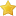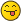• 0 Vote(s) - 0 Average
• 1
• 2
• 3
• 4
• 5
 [Tutorial] Numbering Systems and Conversions Author Message
Hidden DragonAmazingPosts: 63
Joined: Jan 2011
Reputation: 4
 Post: #1 [Tutorial] Numbering Systems and Conversions Though this applies to different languages, I think it is most important in assembly language. We'll take a look at the decimal numbering system, the binary numbering system, and the hexadecimal numbering system. Decimal Numbering System The decimal numbering system is what we use in everyday life. It is operated on base 10. When you think of the number 429 it can be represented different ways. Code: ```429 or 4x10^2 + 2x10^1 + 9x10^0 which gives 400 + 20 + 9 or 429``` Binary Numbering System The binary numbering system is operated on base 2. When you take 429 it can be represented as Code: ```110101101 or 1x2^8 + 1x2^7 + 0x2^6 + 1x2^5 + 0x2^4 + 1x2^3 + 1x2^2 + 0x2^1 + 1x2^0 which gives us 256 + 128 + 0 + 32 + 0 + 8 + 4 + 0 + 1 or 429``` Hexadecimal Numbering System The hexadecimal numbering system is operated on base 16. Some people shorten hexadecimal to hex but that is not the same thing. Hex would denote a base 6 instead of base 16. Since we can only use the numbers 0-9, we also need other characters for 10-15. Instead of making up symbols we use the letters A-F. A=10 B=11...F=15. Again using our 429 example, we have Code: ```1AD or 1x16^2 + 10x16^1 + 13x16^0 which gives us 256 + 160 + 13 which equals 429``` Now we're going to talk about conversion between all of these. Binary to hexadecimal and hexadecimal to binary is the easiest conversion we can do so we're going to start with that. Binary to Hexadecimal Say we have the number 11010. First we have to make our binary number divisible by four. Since we have five numbers we need to fill 3 with 0s. Now we've got 00011010. We have to break that up into four binary digits. 0001 1010 Now that we have that, we can convert to hexadecimal. If you know this conversion chart, it's very easy to convert. [spoiler]Binary Hexadecimal 0000 0 0001 1 0010 2 0011 3 0100 4 0101 5 0110 6 0111 7 1000 8 1001 9 1010 A 1011 B 1100 C 1101 D 1110 E 1111 F[/spoiler] That conversion chart would be good to memorize. Using the chart we have Code: ```0001   1010 1       A 1A.``` Hexadecimal to Decimal Lets use another number for our hexadecimal to decimal numbering. For this, we'll use the number 42B2. This isn't a very hard conversion to do either although none of these really are that difficult. Code: ```42B2 gives us 4x16^3 + 2x16^2 + 11(B)x16^1 + 2x16^0 16,384 + 512 + 176 + 2 17,074.``` Now lets convert our number that we got from binary to hexadecimal. Code: ```1A 1x16^1 + 10x16^0 16 + 10 26.``` Binary to Decimal We're going to use our previous example of 11010 for this problem. This is base 2 so it's also pretty easy to do. Code: ```11010 1x2^4 + 1x2^3 + 0x2^2 + 1x2^1 + 0x2^0 16 + 8 + 0 + 2 + 0 26``` It's pretty easy to reverse all of these formulas and get the opposite starting with the opposite. Hope you've learned something with this. If you have any questions, feel free to post them. Just don't ask about hex to decimal because it's the hardestReally, feel free to ask about anything. Have fun with your conversions!
01-16-2011 03:21 AM
IronsideSite Owner